# Dune Core Modules (2.9.0)

Reference Elements

## Classes

struct  Dune::Geo::ReferenceElements< ctype_, dim >

class  Dune::Geo::ReferenceElement< Implementation >
This class provides access to geometric and topological properties of a reference element. More...

## Typedefs

template<typename... T>
using Dune::Transitional::ReferenceElement = unspecified-type
Returns the type of reference element for the argument types T... More...

template<typename T , int dim>
using Dune::ReferenceElement = unspecified-type
Returns the type of reference element for the argument type T. More...

## Functions

template<typename... T>
unspecified value type Dune::referenceElement (T &&... t)
Returns a reference element for the objects t.... More...

template<typename T , int dim>
auto Dune::referenceElement (const Dune::GeometryType &gt, Dune::Dim< dim >={})
Returns a reference element of dimension dim for the given geometry type and coordinate field type. More...

template<typename T , int dim, std::enable_if_t< IsNumber< std::decay_t< T >>::value, int > = 0>
auto Dune::referenceElement (const T &, const Dune::GeometryType &gt, Dune::Dim< dim >)
Returns a reference element of dimension dim for the given geometry type and coordinate field type. More...

# Introduction

In the following we will give a definition of reference elements and subelement numbering. This is used to define geometries by prescribing a set of points in the space $$\mathbf{R}^w$$.

The basic building block for these elements is given by a recursion formula which assigns to each set $$E \subset \mathbf{R}^d$$ either a prism element $$E^\vert\subset \mathbf{R}^{d+1}$$ or a pyramid element $$E^\circ\subset \mathbf{R}^{d+1}$$ with $$E^\vert = \lbrace (x,\bar{x}) \mid x \in E, \bar{x} \in [0,1] \rbrace$$ and $$E^\circ = \lbrace ((1-\bar{x})x,\bar{x}) \mid x \in E, \bar{x} \in [0,1] \rbrace$$. The recursion starts with a single point $$P_0=0\in\mathbf{R}^0$$.

For $$d=1,2,3$$ this leads to the following elements

• $$d=1$$: $$L_1 = P_0^\vert = P_0^\circ = [0,1]$$ is a line.
• $$d=2$$: $$Q_2 = L_1^\vert$$ is a cube and $$S_2 = L_1^\circ$$ is a simplex.
• $$d=3$$: $$Q_3 = Q_2^\vert$$ is a cube, $$S_3 = S_2^\circ$$ is a simplex, $$\mathrm{pyramid}_3 = Q_2^\circ$$ is a pyramid, and $$\mathrm{prism}_3 = S_2^\vert$$ is a prism.

In general if $$Q_d$$ is a cube then $$Q_d^\vert$$ is also a cube and if $$S_d$$ is a simplex then $$S_d^\circ$$ is also a simplex.

Based on the recursion formula we can also define a numbering of the subentities and also of the sub-subentities of $$E^\vert$$ or $$E^\circ$$ based on a numbering of $$E$$. For the subentities of codimension $$c$$ we use the numbering

• $$E^\vert$$: the first numbers are assigned to the entities parallel to the $$x_d-$$axis in the same order as the subentites of the same codimension in $$E$$; then to the subentities of codimension $$c-1$$ in the bottom followed by those in the top.
• $$E^\circ$$: in this case we first number the subentities of codimension $$c-1$$ in the bottom, followed by each subentity based on a subentity of codimension $$c$$ in $$E$$.

For the subentity of codimension $$cc$$ in a codimension $$c$$ subentity $$E'$$ we use the numbering induced by the numbering the reference element corresponding to $$E'$$.

Here is a graphical representation of the reference elements:

1. One-dimensional reference element. For d=1 the simplex and cube are identical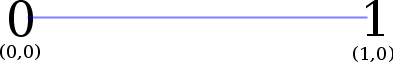2. Two-dimensional reference simplex (a.k.a. triangle)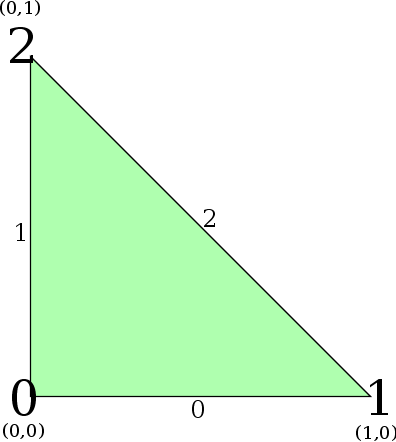3. Three-dimensional reference simplex (a.k.a. tetrahedron)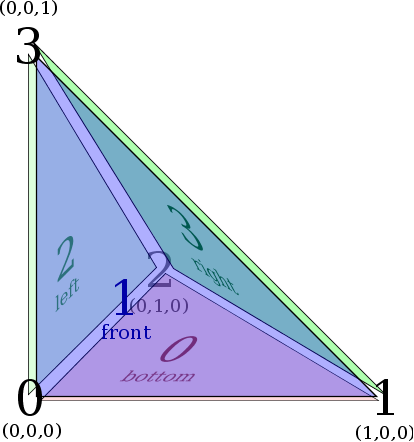Face Numbering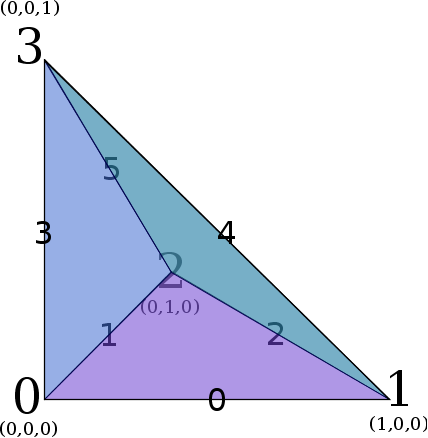Edge Numbering
4. Two-dimensional reference cube (a.k.a. quadrilateral)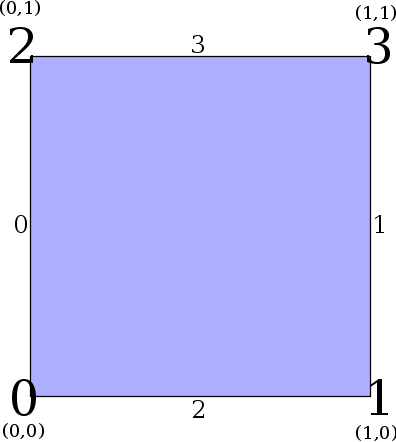5. Three-dimensional reference cube (a.k.a. hexahedron)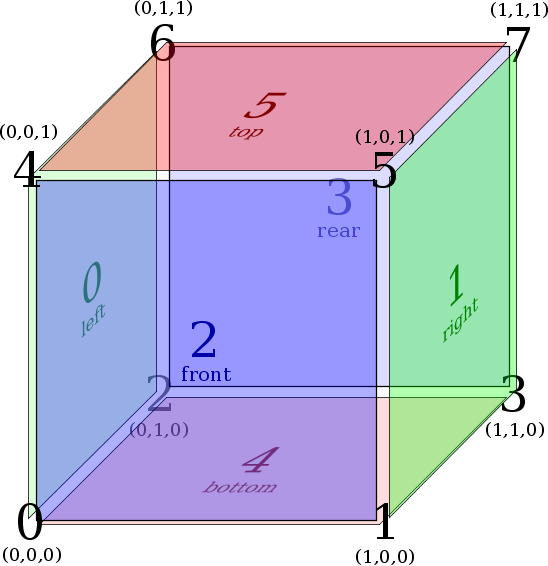Face Numbering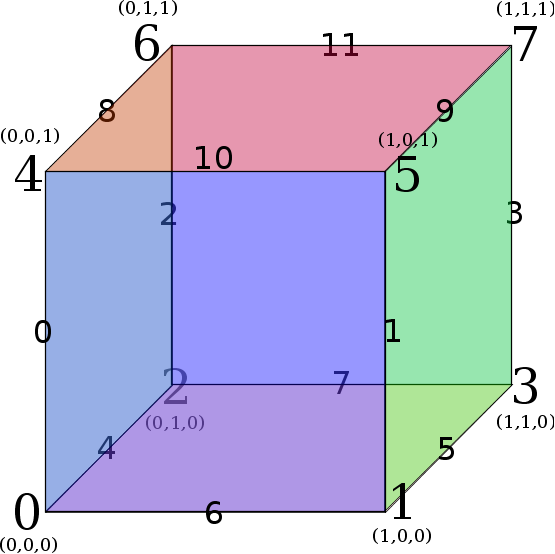Edge Numbering
6. Prism reference element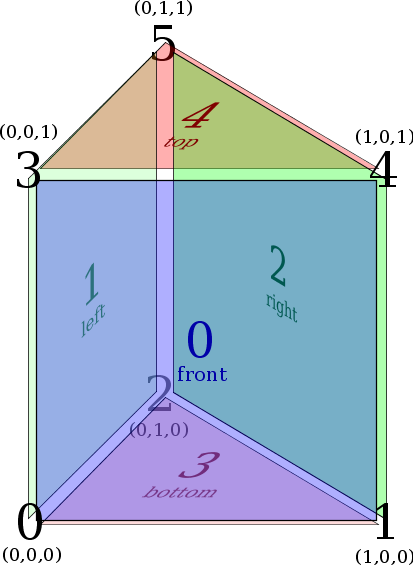Face Numbering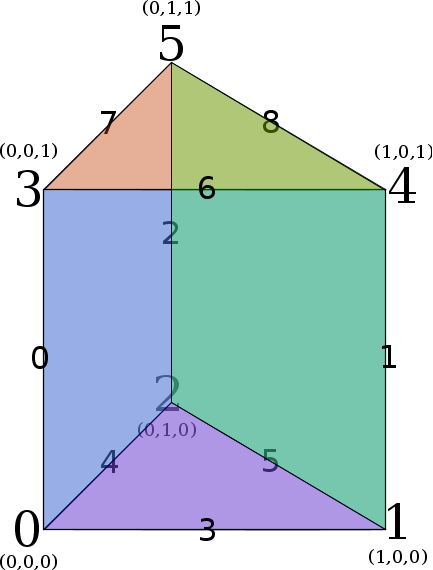Edge Numbering
7. Pyramid reference element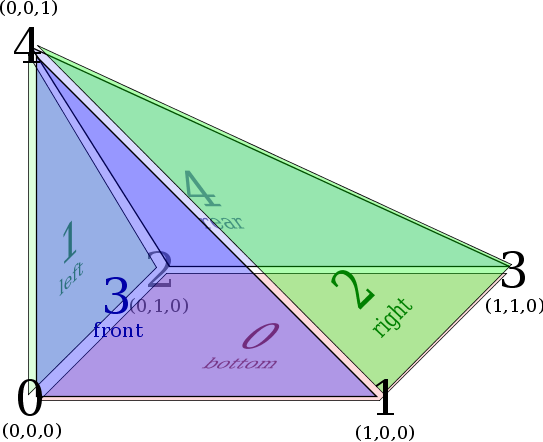Face Numbering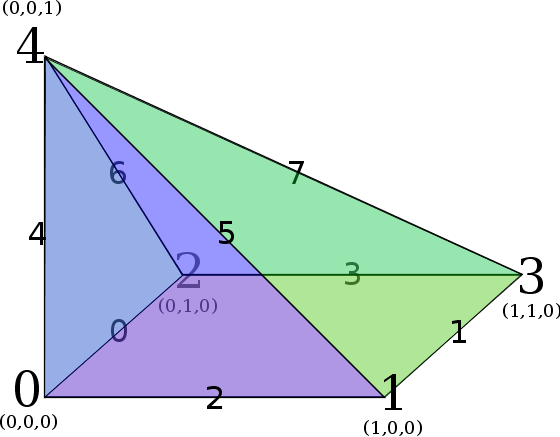Edge Numbering

In addition to the numbering and the corner coordinates of a reference element $$\hat{E}$$ we also define the barycenters $$b(\hat{E})$$, the volume $$|\hat{E}|$$ and the normals $$n_i(\hat{E})$$ to all codimension one subelements.

The recursion formula is also used to define mappings from reference elements $$E$$ to general polytop given by a set of coordinates for the corner points - together with the mapping $$\Phi$$, the transpose of the Jacobian $$D\Phi^T(x)\in\mathbf{R}^{d,w}$$ is also defined where $$d$$ is the dimension of the reference element and $$w$$ the dimension of the coordinates. This suffices to define other necessary parts of a Dune geometry by LQ-decomposing $$D\Phi^T$$: let $$D\Phi^T(x) = LQ$$ be given with a lower diagonal matrix $$L\in \mathbf{R}^{d,d}$$ and a matrix $$Q\in\mathbf{R}^{d,w}$$ which satisfies $$Q Q^T = I$$:

• Jacobian inverse transpose

$D\Phi(x)^{-T}:=Q^T L^{-1}.$

• Integration element

$\sqrt{\mathrm{det}(D\Phi(x)^T D\Phi(x))} = \sqrt{\mathrm{det}(LL^T)} = \Pi_{i=1}^d l_{ii}.$

• Volume

$\int_{\hat{E}} \Pi_{i=1}^d l_{ii}(x) d\;x = |\hat{E}| \Pi_{i=1}^d l_{ii}(b(\hat{E})).$

(Here some assumptions on the degree of the integration element is used.)

The next sections describe the details of the construction.

# Reference Topology

We define the set $$\mathcal{T}$$ of reference topologies by the following rules:

• $$\mathcal{T}$$ contains an element $$\mathbf{p}$$ that we call the point topology.
• For $$T \in \mathcal{T}$$, $$\mathcal{T}$$ contains an element $$T^\vert$$ that we call the prism over $$T$$.
• For $$T \in \mathcal{T}$$, $$\mathcal{T}$$ contains an element $$T^\circ$$ that we call the pyramid over $$T$$.

For each reference topology $$T$$ we define the following values:

• Dimension: The point topology has dimension zero and the dimension of a prism or a pyramid topology over $$t$$ has dimension $$d(t)+1$$.
• Size: For $$c=0,\dots,d$$ with $$d=d(T)$$ we define the number $$s_c(T)$$ through
• $$s_0(T)=1$$.
• If $$T=t^\vert$$ then $$s_d(T)=2s_{d-1}(t)$$ and for $$c\in \{1,\dots,d-1\}$$ we have $$s_c(T)=s_{c}(t)+2s_{c-1}(t)$$.
• If $$T=t^\circ$$ then $$s_d(T)=s_{d-1}(t)+1$$ and for $$c\in \{1,\dots,d-1\}$$ we have $$s_c(T)=s_{c-1}(t)+s_{c}(t)$$.
• Subtopology: Given a reference topology $$T$$ of dimension $$d$$ and a codimension $$c=0,\dots,d$$ we now define the subtopology $$S_{c,i}(T)\in\mathcal{T}$$ for $$i=1,\dots,s_c(T)$$:
• $$S_{0,1}(T)=T$$ and $$S_{d,i}(T)=\mathbf{p}$$
• For $$T=t^\vert$$ and $$c\in \{1,\dots,d-1\}$$ we define using the abbreviations $$p=s_{c}(t),q=s_{c-1}(t)$$

$S_{c,i}(T) = \left\{\begin{array}{ll} S_{c,i}(t)^\vert & \mbox{for}\; i=1,\dots,p, \\ S_{c-1,i-p}(t) & \mbox{for}\; i=p+1,\dots,p+q, \\ S_{c-1,i-p-q}(t) & \mbox{for}\; i=p+q+1,\dots,p+2q. \end{array}\right.$

• For $$T=t^\circ$$ and $$c\in \{1,\dots,d-1\}$$ we define using the abbreviations $$p=s_{c}(t),q=s_{c-1}(t)$$

$S_{c,i}(T) = \left\{\begin{array}{ll} S_{c-1,i}(t) & \mbox{for}\; i=1,\dots,q, \\ S_{c,i-q}(t)^\circ & \mbox{for}\; i=q+1,\dots,q+p. \end{array}\right.$

Notice that the number of vertices (i.e., subtopologies of codimension $$d(T)$$) of a topology $$T$$ does not uniquely identify the topology. To see this, consider the topologies $$T_1 = \mathbf{p}^{\vert\vert\vert\circ\circ}$$ and $$T_2 = \mathbf{p}^{\circ\circ\circ\circ\vert}$$. For these topologies we have $$s_d( T_1 ) = s_d( T_2 ) = 10$$.

# Reference Domains

For each reference topology $$T$$ we associate the set of corners $$\mathcal{C}(T):=(p_i(T))_{1=1}^{s_d(T)}\subset\mathbf{R}^d(T)$$ defined through

• $$T=\mathbf{p}$$: $$p_0(T)=0\in\mathbf{R}^0$$
• $$T=t^\vert$$: $$p_k(T)=(p_k(t),0),p_{d'+k}(T)=(p_k(t),1)$$ for $$k=1,\dots,d'$$, with $$d'=s_d(t)$$.
• $$T=t^\circ$$: $$p_k(T)=(p_k(t),0)$$ for $$k=1,\dots,d-1$$ and $$p_d(T)=e_d$$ with $$d=s_d(T)$$

The convex hall of the set of points $$\mathcal{C}(T)$$ defines the reference domain $$\mathrm{domain}(T)$$ for the reference topology $$T$$; it follows that

• $$\mathrm{domain}( \mathbf{p} ) := \lbrace 0 \rbrace \subset \mathbf{R}^0$$,
• $$\mathrm{domain}( T^\vert ) := \mathrm{domain}( T ) \times [0,1]$$,
• $$\mathrm{domain}( T^\circ ) := \lbrace ((1-\bar{x})x,\bar{x}) \mid x \in \mathrm{domain}( T ), \bar{x} \in [0,1] \rbrace$$.

# Reference Elements and Mappings

A pair $$E=(T,\Phi)$$ of a topology $$T$$ and a map $$\Phi:\mathrm{domain}( T ) \to \mathbf{R}^w$$ with $$w\geq d(T)$$ is called an element.

The reference element is the pair $$E(T)=(T,{\rm id})$$.

For a given set of points $$\mathcal{C}:=(p_i)_{1=1}^{s_d(T)}\subset\mathbf{R}^w$$ we define a mapping $$\Phi_T(\mathcal{C},\cdot)\mathrm{domain}( T ) \to \mathbf{R}^w$$ through $$\Phi_T(\mathcal{C};p_i(T))=p_i$$ for all $$p_i(T)\in \mathcal{C}(T)$$. This mapping can be expressed using the recursive definition of the reference topologies through:

• $$\Phi_{\mathbf{p}}( (p_0) ; x ) = p_0$$,
• $$\Phi_{T^\vert}( (p_1,\ldots,p_{s_d(T^\vert)} ; (x,\bar{x}) ) = (1 - \bar{x}) \Phi_T( p_1,\ldots,p_{s_d(T)} ; x ) + \bar{x} \Phi_T( p_{s_d(T)+1},\dots,p_{s_d(T^\vert)} ; x )$$ with $$x\in \mathrm{domain}( T )$$ and $$\bar{x}\in[0,1]$$.
• $$\Phi_{T^\circ}( p_1,\ldots,p_{s_d(T^\circ)}) ; (x,\bar{x}) ) = (1 - \bar{x}) \Phi_T( p_1,\ldots,p_{s_d(T)-1} ; x ) + \bar{x} p_{s_d(T^\circ)}$$ with $$x\in \mathrm{domain}( T )$$ and $$\bar{x}\in[0,1]$$.

# Numbering of Subelements

Given a reference topology $$T$$, a codimension $$c\in\{0,\dots,d(T)\}$$ and a subtopology $$S_{c,i}(T)$$ we define a subset of the corner set $$\mathcal{C}(T)$$ $$\mathcal{C}_{c,i}(T)= (p_{k_j}(T))_{j=1}^{s_{d(S_{c,i}(T)}(S_{c,i}(T))}$$ given by the subsequence $$\mathcal{K}_{c,i}(T)=(k_j)_{j=1}^{s_{d(S_{c,i}(T)}(S_{c,i}(T))}$$ of $$\{1,dots,s_{d(T)}(T)\}$$:

$$\mathcal{C}_{0,0}(T) = \mathcal{C}(T)$$, $$\mathcal{C}_{d(T),i}(T) = (p_i(T))$$, and for $$c\in\{1,\dots,d(T)-1\}$$ we define $$\mathcal{K}_{c,i}(T)=(k_j)_{j=1}^{s_{d(S_{c,i}(T)}(S_{c,i}(T))}$$ through the recursion

• $$T=t^\vert$$:
For $$i=1,\dots,s_c(T)$$ we define $$\mathcal{K}_{c,i}(T)=(k_1,\dots,k_n, k_1+s_{d(t)}(t)\dots,k_n+s_{d(t)}(t))$$ with $$\mathcal{K}_{c,i}(T)= \{k_1,\dots,k_n\}$$.
For $$i=s_(T)+1,\dots,s_c(T)+s_{c+1}(T)$$ we define $$\mathcal{K}_{c,i}(T)=(k_1,\dots,k_n)$$ with $$\mathcal{K}_{c-1,i}(T)= \{k_1,\dots,k_n\}$$.
For $$i=s_c(T)+s_{c+1}(T)+1,\dots,s_c(T)+2s_{c+1}(T)$$ we define $$\mathcal{K}_{c,i}(T)=(k_1+s_{d(t)}(t),\dots,k_n+s_{d(t)}(t))$$ with $$\mathcal{K}_{c-1,i}(T)= \{k_1,\dots,k_n\}$$.
• $$T=t^\circ$$:
For $$i=1,\dots,s_{c-1}(T)$$ we define $$\mathcal{K}_{c,i}(T)=(k_1,\dots,k_n)$$ with $$\mathcal{K}_{c-1,i}(T)= \{k_1,\dots,k_n\}$$.
For $$i=s_{c-1}(T)+1,\dots,s_{c-1}(T)+s_c(T)$$ we define $$\mathcal{K}_{c,i}(T)=(k_1,\dots,k_n,s_{d(T)}(T))$$ with $$\mathcal{K}_{c-1,i}(T)= \{k_1,\dots,k_n\}$$.

Given these subsets we define subreference elements $$E_{c,i}(T)=(S_{c,i}(T),\Phi_{c,i}(T))$$ of $$E(T)$$ given by the following mapping $$\Phi_{c,i}(T,\cdot)=\Phi(S_{c,i}(T),\mathcal{C}_{c,i}(T),\cdot)$$.

Furthermore we define a numbering of the subreference elements of each subreference element in $$T$$. This is the number $$k=\mathrm{index}_{c,i,cc,ii}(T)$$ for $$c\in\{0,\dots,d(T)\}$$, $$i\in\{1,\dots,s_c(T)\}$$, and $$cc\in\{0,\dots,d(S_{c,i})\}$$, $$ii\in\{1,\dots,s_{cc}(S_{c,i})\}$$ for which

$\Phi_{c,i}(S_{c,i}(T))\circ \Phi_{cc,ii}(S_{cc,ii}(S_{c,i}(T))) = \Phi_{c+cc,k}(T).$

## ◆ ReferenceElement [1/2]

template<typename... T>
 using Dune::Transitional::ReferenceElement = typedef unspecified-type

Returns the type of reference element for the argument types T...

This type alias can be used to get the type of a reference element if you want to store the element as a class member or need to access nested type information. Normally, it will return the type of reference element that a call to referenceElement(T...) would return, for example for a geometry:

Cell::Geometry geo = cell.geometry();
unspecified value type referenceElement(T &&... t)
Returns a reference element for the objects t....
unspecified-type ReferenceElement
Returns the type of reference element for the argument type T.
Definition: referenceelements.hh:497

There is also a special shorthand signature for the default reference elements:

constexpr int dim = ...;
auto geometry_type = ...;
Dune::ReferenceElement<double,Dune::Dim<dim>> = referenceElement<double,dim>(geometry_type);
Dune::ReferenceElement

## ◆ ReferenceElement [2/2]

template<typename T , int dim>
 using Dune::ReferenceElement = typedef unspecified-type

Returns the type of reference element for the argument type T.

This type alias can be used to get the type of a reference element if you want to store the element as a class member or need to access nested type information. Normally, it will return the type of reference element that a call to referenceElement(T) would return, for example for a geometry:

Cell::Geometry geo = cell.geometry();

In the long run, we want to support multiple types T here, but for now that does not work due to backwards compatibility reasons. You can also still obtain the type of a standard reference element using Dune::ReferenceElement<ctype,dim>, but this is deprecated in DUNE 2.6. If you need support for ReferenceElement with multiple type arguments, you can use Dune::Transitional::ReferenceElement for now, which supports multiple types, but not the backwards compatibility mode and will become the default after the release of DUNE 2.6. There is also a special shorthand signature for the default reference elements:

Deprecated:
Using the syntax Dune::ReferenceElement<ctype,dim> is deprecated in DUNE 2.6. You have the following alternatives:
Dune::Transitional::ReferenceElement

## ◆ referenceElement() [1/3]

template<typename T , int dim>
 auto Dune::referenceElement ( const Dune::GeometryType & gt, Dune::Dim< dim > = {} )

Returns a reference element of dimension dim for the given geometry type and coordinate field type.

This function allows you to obtain a reference element for a given coordinate type, dimension and GeometryType:

auto gt = ...;
auto ref_el = referenceElement<ctype,dim>(gt);
auto ref_el = referenceElement<ctype>(gt,Dune::Dim<dim>());
bool gt(const T &first, const T &second, typename EpsilonType< T >::Type epsilon)
test if first greater than second
Definition: float_cmp.cc:158
Static tag representing a dimension.
Definition: dimension.hh:16

## ◆ referenceElement() [2/3]

template<typename T , int dim, std::enable_if_t< IsNumber< std::decay_t< T >>::value, int > = 0>
 auto Dune::referenceElement ( const T & , const Dune::GeometryType & gt, Dune::Dim< dim > )

Returns a reference element of dimension dim for the given geometry type and coordinate field type.

This function allows you to obtain a reference element for a given coordinate type, dimension and GeometryType:

auto gt = ...;
auto ref_el = referenceElement(ctype(),gt,Dune::Dim<dim>());

## ◆ referenceElement() [3/3]

template<typename... T>
 unspecified value type Dune::referenceElement ( T &&... t )

Returns a reference element for the objects t....

The freestanding function referenceElement is a generic entry point for getting reference elements for arbitrary objects that support the operation. As it relies on argument-dependent lookup, the function should be called without any qualifying namespace. Note, however, that the versions of referenceElement() for a dimension and GeometryType with explicit template arguments cannot be found by ADL, so you have to explicitly pull them in or qualify the call using Dune:::

{
// option 1: using
auto ref_el = referenceElement<double,dim>(geometry_type);
}
{
// option 2: explicitly put in Dune::
auto ref_el = Dune::referenceElement<double,dim>(geometry_type);
}
{
// option 3: use version without explicit template arguments
auto ref_el = referenceElement(double(),geometry_type,Dune::Dim<dim>());
}

The returned object is guaranteed to have value semantics, so you can copy it around and store it by value.

The grid geometries in dune-grid support this function, and thus most people will use this function as

for (const auto& cell : elements(grid_view))
{
auto geo = cell.geometry();
auto ref_el = referenceElement(geo);
// do some work...
}

This does of course also work for entities of other codimensions.|  Legal Statements / Impressum  |  generated with Hugo v0.80.0 (Mar 19, 23:30, 2023)